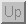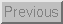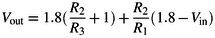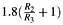Next: Additional Signal Conditioning Circuits Up: Capacitance to Voltage Previous: Circuits

### Examples

ADXL50 Accelerometer

The ADXL50 accelerometer provides as its output a voltage level which varies in proportion to the amount of acceleration experienced along its sensitive axis. It is calibrated such that under no acceleration the output will be 1.8 volts. Furthermore, an acceleration of 50g (where 1g is the acceleration due to gravity) will cause a voltage swing of 1volt (ie. an acceleration of 50g would result in an output of either 2.8volts, or .8 volts depending upon the direction). In many cases this voltage swing would not be appropriate for a specific application, hence one must use a circuit which converts the output voltage into a more useful voltage range. For example, one might desire a more sensitive device which varied 1volt for every g of acceleration. Figure 39 depicts a circuit which accomplishes this task.Figure 39: Schematic of an op-amp circuit which converts the output voltage of the ADXL-50 to a range which is suitable for analog-digital conversion.

The output voltage of this circuit is given by:When no acceleration is applied to the device, the output voltage will be 1.8volts which makes the second term will be zero, and the output will be, the new zero-g output. The output voltage can be written as:whereis the deviation of the output voltage from 1.8 volts. A 50g acceleration will result inof 1volt, which will be scaled by. In this way, we see thatdetermines the sensitivity of the output, while the new zero-g output is determined by.

Tim Stilson
Thu Oct 17 11:25:23 PDT 1996